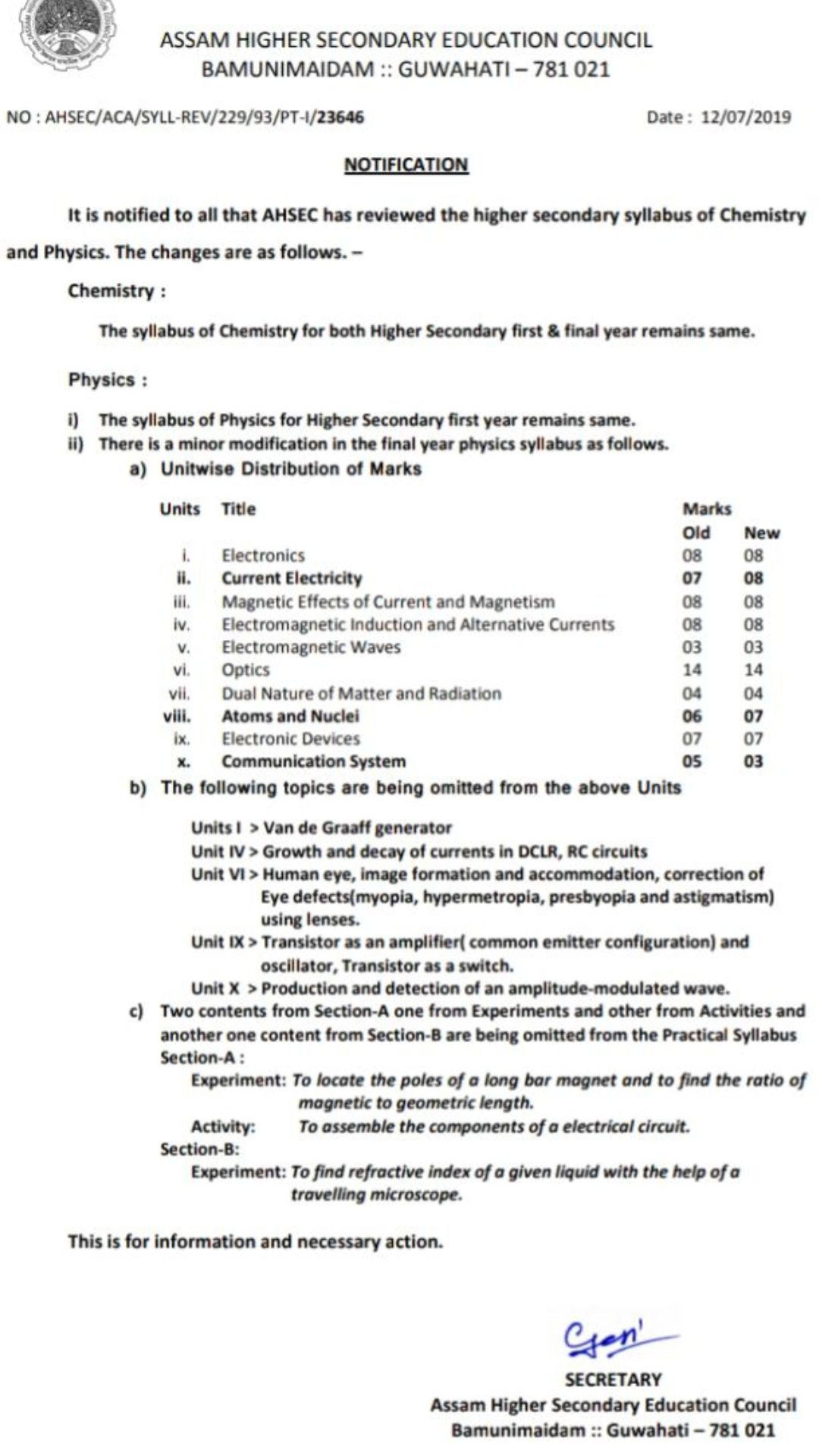## Practice paper for AHSEC class 12 on Magnetic effect and Magnetic properties

Total marks 25    Total time-1hour

Set A (each question carries one mark)

01. What is 1 tesla?

02. Give the dimensional formula of permeability

03. What is the resonance condition of  cyclotron?

04. What is an ideal voltmeter?

05. State gauss law in magnetism.

Set B (its question carries 2 marks)

01. How a galvanometer can be converted into an ammeter? Explain with diagram

02. Define the magnetic elements of earth

03. Find expression for torque acting on a bar magnet placed in a uniform magnetic field

04. Give the difference between diamagnetic and paramagnetic substance

Set C(Each question carries 3 marks)

01. Using ampere’s circuital law find expression for magnetic field due to solenoid.

02. Find expression for magnetic field at the axial point of  a current loop

03. Find the expression for force acting between two parallel current carrying conductor in the same direction.

04. Find expression for magnetic moment due to an revolving electron what is Bohr magneton?

## Practice paper for class 12 AHSEC : 2020

1. Total marks-25  Total time-1hour

Set A(Each question carries 1 mark)

01. Write down the properties of electric charge

02. Draw the diagram of electric lines of force due to thin sheet of charge

03. What is an equipotential surface?

04. Give the resistance of a resistor with colour code of red black green silver

05. How mobility of charge carriers is related to temperature?

Set B(Each question carries 2 marks)

01. Give the difference between polar and nonpolar dielectrics.

02. find the expression for torque acting on an electric dipole placed in a uniform electric field.

03. Explain the two laws of kirchoff laws with diagram

04 . Find the expression for capacitance of parallel plate capacitor

Set C(each question carries 3 marks)

01. Express coulomb’s law in vector form

02. Using gauss theorem find an expression for electric field due to the hollow sphere at a point outside and  on its surface

03. Find expression for electric potential due to an electric dipole at any point

04. Using the concept of drift velocity establish ohm’s law

## Practice paper for AHSEC class 12 of 2020

Total mark -36

Total time -90 minutes

Each question carries carries 3 marks

Q1- Obtain the relation between angle of minimum deviation for a prism and its refractive index .

Q2- Draw the ray diagram for reflecting type telescope . Write down advantages of reflecting type telescope over refracting type telescope .

Q3- define critical angle  . Write down the condition for total internal reflection .What is optical fibre .

Q4-Write down Huygens principles . Draw the diagram for wavefront  due to a point source.

Q5- Prove that for destructive interference path difference between the two light waves should be odd integral multiple of λ/ 2.

Q6- State Malus law . Prove that when light is incident at polarising angle on a reflecting surface angle between reflected and refracted light is 90 º

Q7- Draw a ray diagram for Davission and Germer  experiment . Write down few uses of de Broglie’s hypothesis.

Q8- State Bohr’s postulate  . Write down its failures .

Q9- Draw and explain binding energy curve.

Q10-  Explain the working of zener diode as voltage regulator

Q11- Give the Boolean expression and truth table of NAND gate . How OR gate can be obtained from NOR gate and NAND gate ?

Q12- Define the following terms :-  noise , attenuation and modulation .

## SAMPLE PAPERS / 2019 / AHSEC / XII / PHYSICS / NO.-01

SAMPLE PAPER 2019_A

## Marking pattern for AHSEC Physics ,2019

Assam higher secondary education council announced new marking pattern for the subject physics 2019 . Marking pattern will be same for unit 1 ,unit 3 , unit 4, unit 5, unit 6, unit 7 and unit 9 . For unit 2 and unit 8 , 1 mark is increased  and for unit 10 2 mark is  decreased . Full marking scheme is given below

.ncert_xi_11